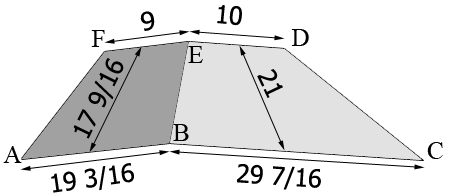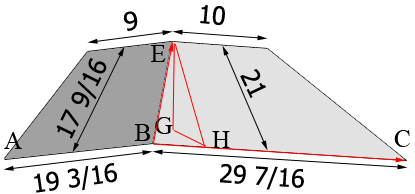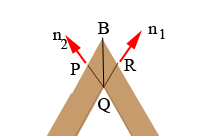SEARCH HOMEMath Central Quandaries & QueriesQuestion from Chuck: I'm building a custom range hood and can't seem to get the angles correct where the front and side panels intersect. I saw a similar post but there's no way I can do the calculations for a Wolfram Alpha! Here are the dimensions that I have (in inches)... Base - Front 29 7/16" x side 19 3/16" Top - Front 10" x side 9" Front Panel Length (from base to top on an angle) 21" Side Panel Length (from base to top on an angle) - 17 9/16" Vertical distance from the base to the top 14 1/4" Any help finding the bevel/miter of the two front corners where the sides meet the front panel would be greatly appreciated. I will need the angle for the saw in degrees. Thank you in advance! Regards, ChuckHi Chuck,

I drew a diagram from my reading of your description. All the dimensions are in inches.The points $A, B$ and $C$ lie in a base plane and the points $D, E$ and $F$ lie in a top plane parallel to the base plane. The vertical distance between these two planes is 14 1/4 inches.

I redrew the diagram with a few extra points labeled. Imagine that I put a Cartesian coordinate system in the diagram with the origin at $B$, $BC$ on the X-axis, $BA$ on the Y-axis and the Z-axis upwards.The point $G$ is on the base plane (the XY plane) directly below $B$ and $EH$ is perpendicular to the X-axis. Thus the length of $EH$ is 21 inches and the length of $EG$ is 14.25 inches. The angle $EGH$ is a right angle and hence using Pythagoras Theorem I get the length of $GH$ to be 15.43 inches. This is the Y-coordinate of the point $E.$ In a similar fashion I found that the X-coordinate of $E$ is 10.27 inches. Hence the vector $BE$ can be written $(10.27, 15.43, 14.25).$ The vector $BC$ can also be written $(29 7/16, 0, 0).$

The cross product of the vectors $BC$ and $BE$ gives a vector perpendicular to the plane containing $B, C, D$ and $E$ (the front panel) which can be normalized to length 1 to obtain a unit vector $n_1} perpendicular to the front panel. I used the command Cross({29 7/16, 0, 0},{10.27, 15.43, 14.25}) in Wolfram Alpha to make this calculation and then normalized the result to obtain $n_1 = (0., -0.678458, 0.734639)$ In a similar fashion I obtained $n_2 = (-0.811264, 0., 0.58468)$ a unit vector perpendicular to the side panel. Now look at the point$B$from below.The angle$PQR$is the angle between$n_1$and$n_2$can be found again using Wolfram Alpha with the command Arccos({-0.811264, 0., 0.58468}.{0., -0.678458, 0.734639})*180/Pi The result is that the measure of the angle$PQR$is 64.5623 degrees, and hence the measure of the angle$BQR$is$\frac{64.5623}{2}= 32.2812$degrees and the measure of the angle$RBQ$is$90 - 32.2812 = 57.7186\$ degrees.

Harley* Registered trade mark of Imperial Oil Limited. Used under license.Math Central is supported by the University of Regina and the Imperial Oil Foundation.